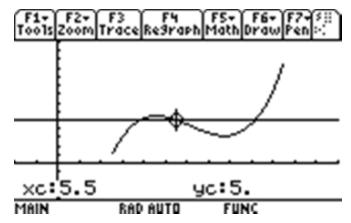# Activities

••• ##### Subject Area

• Math: Calculus: Derivatives
• Math: Calculus: Other Functions

• ##### Author9-12

15 Minutes

• ##### Device
• TI-89 / TI-89 Titanium
• ##### Report an Issue

"Value"able Theorems#### Activity Overview

Students are introduced to the Intermediate Value Theorem and Extreme Value Theorem from a graphical perspective.

#### Key Steps

•This activity is a teacher led demonstration of the Intermediate Value Theorem and the Extreme Value Theorem from a graphical perspective. Students may complete the accompanying worksheet if the teacher desires. The function y1(x) is a continuous function on a finite closed interval [a, b]. The teacher can move a horizontal line up and down to change “k” and graphically demonstrate that there is a value c on the interval [a, b] such that y1(c) = k.